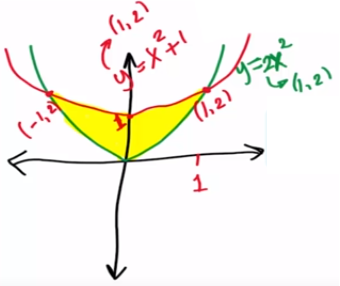Need Help?

Subscribe to Calculus C

###### \${selected_topic_name}
• Notes

Evaluate $\iint_{D}(x+2 y) d A,$ where $D$ is the region bounded by the
parabolas $y=2 x^{2}$ and $y=1+x^{2}$${\int_{x_{1}=-1}^{x_{2}=1}}_{R} \left[\int_{y_{1}=2x^{2}}^{y_{2}=x^{2}+1}(x+2 y)d y\right] d x$

$=\int_{x_{1}=-1}^{x_{2}=1} \int_{2 x^{2}}^{x^{2}+1}\left[{xy+y^{2}}\right] d x=\int_{-1}^{1} \left[x\left(x+1-2 x^{2}\right)+\left(x^{2}+1\right)^{2}-\left(2 x^{2}\right)^{2}\right]$

=$\int_{-1}^{1} \left(x-x^{3}\right)+x^{4}+2 x^{2}+1-4 x^{4}$

$\int_{-1}^{1} -3 x^{4}-x^{3}+2 x^{2}+x+1$

$=\frac{32}{15}$